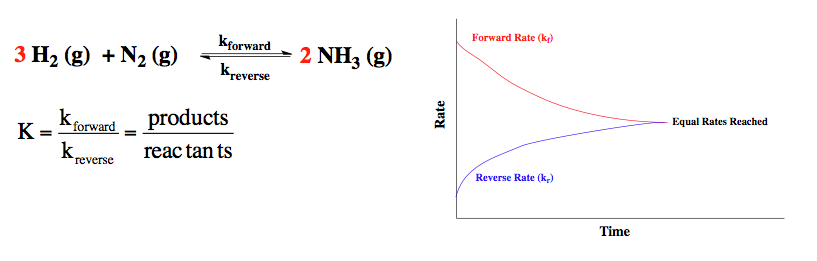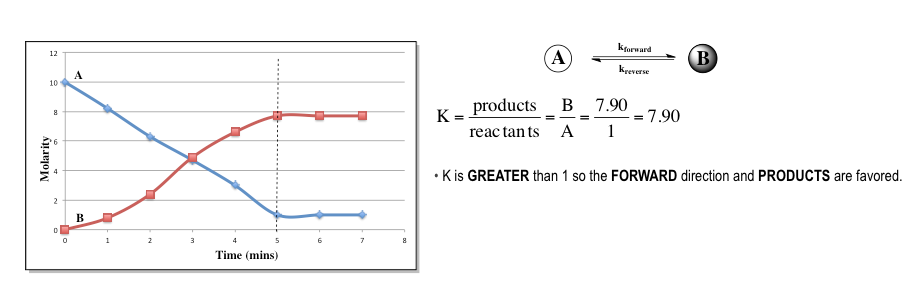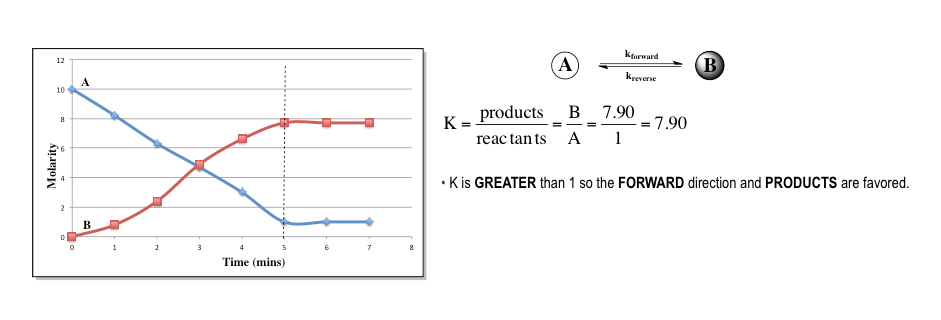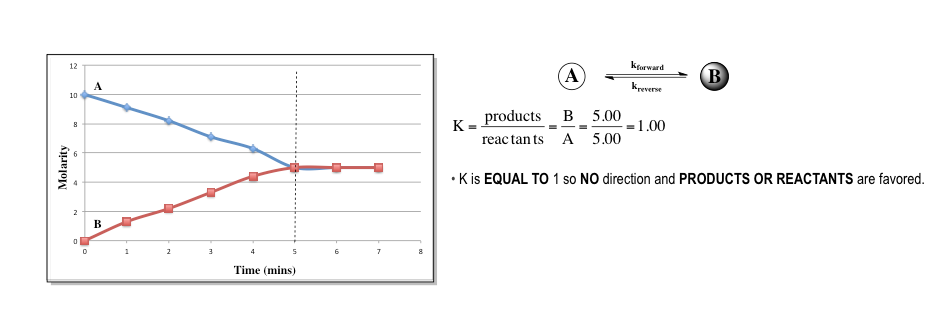Ch.6 - Chemical EquilibriumWorksheetSee all chapters
 Ch.1 - Chemical Measurements 2hrs & 10mins 0% complete Worksheet Ch.2 - Tools of the Trade 1hr & 26mins 0% complete Worksheet Ch.3 - Experimental Error 1hr & 51mins 0% complete Worksheet Ch.4 + 5 - Statistics, Quality Assurance and Calibration Methods 2hrs & 1min 0% complete Worksheet Ch.6 - Chemical Equilibrium 3hrs & 43mins 0% complete Worksheet Ch.7 - Activity and the Systematic Treatment of Equilibrium 1hr & 1min 0% complete Worksheet Ch.8 - Monoprotic Acid-Base Equilibria 1hr & 52mins 0% complete Worksheet Ch.9 - Polyprotic Acid-Base Equilibria 2hrs & 16mins 0% complete Worksheet Ch.10 - Acid-Base Titrations 2hrs & 22mins 0% complete Worksheet Ch.11 - EDTA Titrations 1hr & 37mins 0% complete Worksheet Ch.12 - Advanced Topics in Equilibrium 1hr & 18mins 0% complete Worksheet Ch.13 - Fundamentals of Electrochemistry 2hrs & 27mins 0% complete Worksheet Ch.14 - Electrodes and Potentiometry 55mins 0% complete Worksheet Ch.15 - Redox Titrations 1hr & 14mins 0% complete Worksheet Ch.16 - Electroanalytical Techniques 1hr & 23mins 0% complete Worksheet Ch.17 - Fundamentals of Spectrophotometry 55mins 0% complete Worksheet BONUS: Chemical Kinetics Not available yet

# The Equilibrium State

See all sections

Under an equilibrium state the rates of the reverse and forward reaction are equal.

###### The Equilibrium State

Concept #1: Most chemical reactions do not go to completion and instead reach a state of chemical equilibrium.

Equilibrium is reached when the rates of the forward and reverse reaction are equal.The magnitude of K determines which direction is favored at equilibrium. If K is greater than 1 then products are favored over reactants.If K is less than 1 then reactants are favored over products.If K is equal to 1 then both reactants and products are favored.###### Equilibrium State Calculations

Example #1: For the following chemical reaction N2 (g) + O2 (g)  2 NO (g), Kc = 3.7 x 10-5, kf = 2.5 x 10-3 and kr = 67.57. Addition of a catalyst increases the forward rate constant 1.8 x 10-1. What is the new reverse rate constant after the addition of the catalyst?

Example #2: Consider the following reactions at 25°C:

Reaction                                                                      Kc
2 NO (g) ⇌ N(g) + O(g)                             1 × 1030
2 H2O (g) ⇌ 2 H(g) + O(g)                       5 × 10−82
2 CO (g) + O(g) ⇌ 2 CO(g)                      3 × 1091

Which compound is most likely to dissociate and give O(g) at 25°C?

a) CO2                          b) NO                           c) CO                           d) H2O

# Practice: Write the equilibrium expression for the following reaction.4 NH3 (g) + 3 O2 (g) ⇌  2 N2 (g) + 6 H2O (l)

Example #3: When reaction 1 and 2 below are added together, the result is reaction 3.

1)  H2O (l) + HNO(aq)  H3O+ (aq) + NO2 (aq)              K1 = 4.50 × 104

2)  H3O+ (aq) + OH (aq)  2 H2O (l)                                        K2 = 1.00 × 1014

3)  HNO(aq) + OH (aq)  NO2 (aq) + H2O (l)                 K3 = ?

Find the equilibrium constant, K3.

A) 4.50 × 1018        B) 2.22 × 1017​        C) 4.50 × 1010​        D) 1.00 × 1014